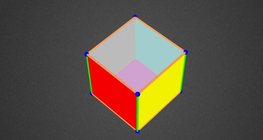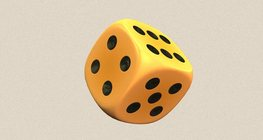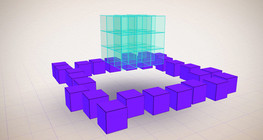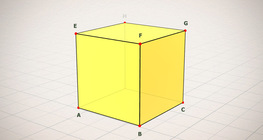Quantity: 0

Total: 0,00

# Nets of a cuboid (exercises)

### Nets of a cuboid (exercises)

This animation demonstrates the different nets of a cuboid and includes a game.

Mathematics

Keywords

net of a cuboid, net, cuboid, solids, exercise, geometry, mathematics

### Related items#### Cuboid

A cuboid is a polyhedron with six rectangular faces.#### Grouping of cuboids

This animation demonstrates various types of cuboids through everyday objects.#### Net of a cube (exercises)

Not all nets consisting of 6 congruent squares are foldable into cubes.#### Coloring a cube

Coloring the vertices, edges and faces of a given cube according to the criteria specified in the exercise.#### Cube

This animation demonstrates the components (vertices, edges, diagonals and faces) of the cube, one of the Platonic solids.#### Cube of cubes

An exercise about the regular hexahedron built from unit cubes to help deepen your knowledge of cubes.#### Dice

Regular dice can be used for solving statistical and probability exercises.#### Notable products

A spectacular demonstration of algebraic expressions.#### Shape games

Find views and nets of three-dimensional shapes.#### Volume and surface area (exercise)

An exercise about the volume and surface area of solids generated from a ´base cube´.#### Constructing shapes (3D)

Building 3D shapes into a given cube mesh with the help of several views.#### Cube (exercises)

Edges, diagonals and faces of a cube can be identified by its vertices.#### Cube puzzle

Building cubes shown in several views from the available unit cubes aids spatial vision and other skills.#### Cube sections (exercise)

Examining solids formed by the intersection of a cube and a plane.#### Building shapes (multi color)

Build 3D shapes from unit cubes with the help of several views.#### Building shapes (one color)

Build 3D shapes from unit cubes with the help of several views.